﻿ A Comparative Study of Meta-heuristics Algorithms in evaluation of Economic Load Dispatch Problems in Power Generating Station with Matlab Codes

# A Comparative Study of Meta-heuristics Algorithms in evaluation of Economic Load Dispatch Problems in Power Generating Station with Matlab Codes

Osaremwinda OP*, Nwohu MN and Kolo JG
Department of Electrical and Electronics Engineering, Federal University of Technology Minna, Nigeria
*Corresponding Author: Osaremwinda OP, Department of Electrical and Electronics Engineering, Federal University of Technology Minna, Nigeria, Tel: 2348023098495, Email: [email protected]

Received Date: Sep 22, 2017 / Accepted Date: Dec 05, 2017 / Published Date: Dec 12, 2017

### Abstract

This paper presents a comparative study of metaheuristics algorithms in evaluation of economic load dispatch problem in power generating station with MATLAB codes. In this paper, the formulation of the ELD problems using mathematical illustrations and MATLAB codes were presented. This consists of the ELD cost model, Model calculations, cost function and parse solution. It also presents the application of some metaheuristics algorithms (solution algorithms) such as ACSA and PSO in solving ELD problem with MATLAB codes. The ELD problem was modeling using Egbin thermal power station, Nigeria as our case study.

Keywords: Economic Load Dispatch (ELD); Ant Colony Search Algorithms (ACSA); Particle Swarm Optimization (PSO); MATLAB codes

#### Introduction

Economic Load Dispatch (ELD) is the reduction of the total cost of power generation (which includes fuel consumption and operational cost) of power generating plants while meeting the various loads demand and power losses in the power transmission system. The objective is to apportion the total load demand and total loss among the various generating units at the same time satisfying the system constraints with reduced generation costs. With Economic Load Dispatch, it is expected that the power utilities plan and forecast optimal load dispatch. Several considerations are made during energy scheduling, these amongst others are to find out the existing generating units, the distance between load centers and the generating units, identifying the operating limits of each generating units such as the ramp rate limits, maximum and minimum generation level, prohibited zones .

Economic Load Dispatch are usually influence by factors such as high operating cost (fuel cost) and transmission losses. The Economic Load Dispatch requires the generation facilities to plan and forecast optimal energy dispatch. Hence the concept is the optimal selection of the generating units in such an economic manner that the total cost of supplying the dynamic requirements of the system is minimized .

Formulation of ELD problem

The ELD problem is an optimization problem that determines the power output of each generating units that will amount to the lowest fuel cost.

Minimize C(x,u) (1)

Subject to:

G(x,u)=0 (2)

H(x,u) ≤ 0 (3)

Where, C=cost function,

x=vector of dependent variables (generating cost)

u=vector of control variables (generator outputs)

G(x,u)=set of non-linear quality constraints (power balance)

H(x,u)=set of inequality constraints (limits in generator outputs).

The objective of the Economic Load Dispatch is to minimize the total operating cost of a power system by adjusting the power output of each of the generators connected to the grid, while satisfying the total load demand plus transmission losses within generator limits.

The generator cost curves are modeled with smooth quadratic (objective) function given by eqn. (4) subject to the equality (power balance) and inequality (generation limits) constraints in eqns. (5) and (7) respectively .

Minimize the objective function,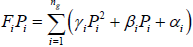(4)

Subject to power balance equation (equality constraint)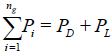(5)

The system losses can be determined by means of a power flow equation solution which is expressed in krons loss formula in eqn. (6)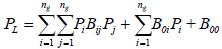(6)

And the (generating limits) inequality constraints,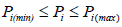(7)

Where FiPi is the total generation cost, αi, βii are cost coefficients, PD is the load demand, Pi is real power generation of unit i, Pj is real power generation of unit j, PL is power transmission loss, ng is number of dispatchable generating plants, Pi(min) and Pi(max) are the minimum and maximum power generation limits respectively also Bij, Boi and Boo are B-coefficient .

Operating cost of a thermal plant

In economic scheduling of different generating plants, the total operating cost is minimal in a Thermal Plant . This generally comprises of the input to the thermal power plant which is expressed in Btu/h or Kcal/h and the output in MW.

The input-output curve of a thermal unit is regarded as heat-rate curve and it is usually a graph drawn between fuel input in Btu/h or kcal/h and power output in MW on the x-axis and y-axis, respectively [7-9].

A typical heat-rate curve for a thermal unit is given in Figure 1, while Figure 2 shows the ordinate of heat-rate curve from Btu/h to ₦/h results in the fuel-cost curve.

Hence, the fuel cost of generator i can be written as a quadratic function of real power generation as shown in eqn. (8).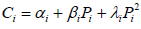(8)

Incremental Fuel-cost curve is obtained by plotting the derivative of the fuel-cost curve versus the real power as shown in Figure 3. It is a measure of how costly it will be to produce the next increment of power. It is drawn by taking the incremental fuel cost in N/MWh as an input on the y-axis and real power in MW as output on the x-axis.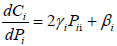(9)

The total operating cost includes the fuel cost, the labor cost and maintenance .

#### Research Methodology

Economic Load Dispatch problem was formulated using mathematical illustrations and MATLAB codes.

The ELD problem is formulated as minimizing a scalar objective function through the optimal operation of a vector of controls parameters. This is mathematically illustrated in eqns. (1-3) and diagrammatically represented in Figure 4 .

The approach consists of two main components: the controlling device and the controlled device are shown in Figure 4. The ELD problem is one that involves the optimal set of generating units. This minimizes the operating cost (mainly fuel cost). The controlled device is the generating cost model, while the solution algorithm (SA) is the controlling device. A vector of generators output is inputted into the generation cost model, which produces a scalar cost of generating those power outputs. The generation cost is passed on to the solution algorithm to be reduced, an iterative process which continues until either a best cost lesser than acceptable minimum is achieved or number of maximum iterations is reached. Figure 5 show a flow diagram for ELD optimization using Solution Algorithms .

Formulation of economic load dispatch problem

The ELD problem is an optimization problem with an aim to find the optimal combination of committed online power generators that will reduce the total operating cost to meet the total system’s power demand while satisfying equality (power balance) and inequality (generation limits) constraints. This is usually done over a period of one hour. The constraints in a practical generator include minimum and maximum generation limits, power balance, ramp rate limits and prohibited operating zones.

For a thermal generating station, the unit fuel cost is shown in the quadratic form in Figure 2, and the goal is to minimize the total operating cost as in eqns. (10) and (11), subject to the generating limit constraint of eqn. (14), power balance constraint of eqns. (12), ramprate limits constraints of eqn. (15), and prohibited operating zones constraints of eqns. (16-18).

Considering a thermal power station of ith generating units G1, G2,…….Gi delivering powers Pg1, Pg2,…..,Pgi respectively connected to a transmission network as shown in Figure 6. Where PD is the total power demand and PL is the total power losses.Each unit has its cost function Ci. The task here is to find the combination of the real power generation for all units such that the total generation cost CT is minimized .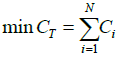(10)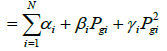(11)

Subject to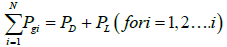(12)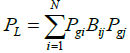(13)

Subject to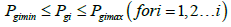(14)

Where CT is the total generation cost, Ci is the generation cost of ith unit αi, βi, γi are cost coefficients, PD is the load demand, Pgi is real power generation of unit i, Pgj is real power generation of unit j, PL is power transmission loss, N is number of dispatchable generating plants, Bij is B-coefficient, P(i(min)) and P(i(max)) are the minimum and maximum power generation limits respectively.

Considering also the ramp-rate limits and prohibited zone, eqn. (14) is modified as eqn. (15),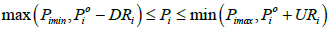(15)

Where Pio is the previous output, Pi is the present output, DR and UR are the down and up ramp-rate limits

The prohibited zones are described by the following inequality constraints .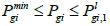(16)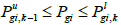(17)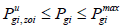(18)

Where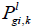and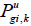are lower and upper bounds of the kth prohibited zone of unit I respectively, k is the index of prohibited zone, zoi (Tables 1 and 2).

Gen units (α) (N/h) (β) (N/MWh) (γ) (N/MW2 h) Pmin Pmax
1 2131.1667 13.1 0.186 55 220
2 2131.1667 13.1 0.186 55 220
3 2131.1667 13.1 0.186 55 220
4 2131.1667 13.1 0.186 55 220
5 2131.1667 13.1 0.186 55 220
6 2131.1667 13.1 0.186 55 220

Table 1: Generator data of Egbin Thermal power station (six generating units) .

B-coefficient data
0.00099 0.00016 -0.0002 -0.0002 -0.0002 0.00015
0.00014 0.00012 -0.0001 -0.0001 -0.0001 0.00012
-0.0001 -0.0001 0.00013 0.00013 0.00012 -0.0001
-0.0001 -0.0001 -0.0001 0.00014 0.00013 -0.0001
-0.0001 -0.0001 0.00013 0.00013 0.00012 -0.0001
0.00015 -0.0001 -0.0001 -0.0001 -0.0001 0.00012

Table 2: Power Losses (B- coefficient) .

Using the Matlab codes , the ELD was modeled from the concept of ELD problem defined in eqn. (10) through to eqn. (18) above showing the power demand, minimum and maximum generating limits, cost coefficients, ramp-rate limits, prohibited zone and B-coefficient. This is model as a function and called Create Model ( ) as shown Figures 7 and 8.

Applying eqns. (10-18), The Model Calculation for the Economic Load Dispatch problem was formulated and the Load apportioned to various generating units (Pgi), Total generated power (PTotal), Total generation cost (CTotal) and Total power losses (PL) were calculated as shown in Figures 9 and 10.

Optimization approach

A main task in the optimization process is constraints handling. The application of the solution algorithm (SA) to solving constrained problems in the ELD involves various techniques of handling constraints, to keep the control variables in feasible region, where all the constraints are satisfied. A technique for handling these constraints constitutes the efficiency within the solution Algorithms to solving this ELD problem .

#### Simulation Approach (Application Of Solution Algorithms To Solve ELD)

The modeling and program development of Solution Algorithm and the ELD problem were accomplished by using MATLAB R2008b software. All program were ran on Intel (R) 2.60 GHz CPU, 2 GB RAM, Window 7 Computer and the modeled ELD was used to evaluate the effectiveness and efficiency of these algorithms (ACSA and PSO), the case study involving six generating units (Egbin thermal station) were applied.

The objective was to minimize the total operating cost, while satisfying the system constraints under the allowable limits.

The network data (Generator data & B-coefficient) were obtained  which were presented in Tables 1 and 2.

The outputs results of the optimization tools which were evaluated are as follows;

• Loads apportioned to the various generating units

• Total Generated Power

• Total Power Loss

• Total Generation Cost.

Application of solution algorithms in solving ELD problem in MATLAB codes

The Create Model, Cost Function modeled in Figures 7 and 8 are called into the solution Algorithms as shown in Figures 11 and 12 for ACSA, and PSO, respectively.

#### Results

Results from simulation using Power Demand of 600 MW (comparison between PSO and ACSA) is shown in Table 3.

Gen Outputs ACSA PSO
G1 84.723 82.348
G2 103.562 103.356
G3 103.894 106.174
G4 108.181 105.734
G5 97.475 97.865
G6 102.176 104.654
Power Demand(MW) 600.000 600.000
Total Power Generated(MW) 600.011 600.130
Total Power Losses 0.011 0,130
Total Generation Cost 31870.672 31891.680

Table 3: Results from simulation using Power Demand of 600MW (comparison between PSO and ACSA).

#### Discussion Of Results

Table 3 shows that the ACSA has effectively reduced the operating cost by 0.07% as compared with that of PSO.

#### Conclusion

The ELD problem has been successfully modeled and the Solution Algorithm applied to the modeled ELD problem in power generating station in this paper. Subsequently, results shows that ACSA has effectively minimized the operating cost as compared to that of PSO.

#### References

Citation: Osaremwinda OP, Nwohu MN, Kolo JG (2017) A Comparative Study of Meta-heuristics Algorithms in evaluation of Economic Load Dispatch Problems in Power Generating Station with Matlab Codes. J Electr Electron Syst 6: 245. DOI: 10.4172/2332-0796.1000245

Copyright: © 2017 Osaremwinda OP, et al. This is an open-access article distributed under the terms of the Creative Commons Attribution License, which permits unrestricted use, distribution, and reproduction in any medium, provided the original author and source are credited.

Select your language of interest to view the total content in your interested language

##### Recommended Journals
Viewmore
###### Article Usage
• Total views: 2623
• [From(publication date): 0-2017 - Dec 16, 2019]
• Breakdown by view type
• HTML page views: 2406Can't read the image? click here to refresh